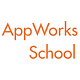# 我想學程式，但到底該從哪個語言入門？

## 程式麻瓜的程式知識課（一）

「我就是看不懂專業術語才要學啊，可不可以先給我看一些人話！」

# Python

## 好理解：

`print(“Python 好棒棒”)`
`#include <iostream.h>int main(){cout << “C++ 好棒棒” << endl;}`
`註* Cout 唸作 c out，不是唸作庫特。`

## 好偷懶：

`int x = 3;`
`x = 3`

## 好作弊：

`#擷取前人的智慧from sklearn.linear_model import LinearRegression#把前人的智慧放進程式碼regressor = LinearRegression()#把自己的資料丟進去，看著魔法發生，讓模組處理好所有事情regressor.fit(X_data, y_data)`
`#擷取前人的智慧 againimport matplotlib.pyplot as plt#設定自己的資料用紅點點標示好plt.scatter(X_data, y_data, color = ‘red’)#設定 regressor 裡面處理好的回歸分析＋預測用一條藍色的線標示好plt.plot(X_data, regressor.predict(X_data), color = ‘blue’)#把圖秀出來plt.show()`
`註* 這邊的程式碼省略了資料前處理以及真正在做回歸時應該要分成 train set / test set 的部分，但大致上使用模組的感覺就是這樣的簡單（但行有餘力還是可以多去了解模組是如何運作的呦）。`

# C++

## Python 跟 C++ 的比較

「C++省電腦的精力，Python 省人類的精力。」

# 學習資源

Written by

Written by

## 胡程維｜Cheng-Wei Hu

#### Voracious learner | Software developer | Finance student | https://fb.me/HcwXd | https://hcwxd.github.io/